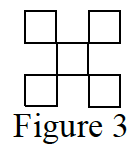### Home > GB8I > Chapter 4 Unit 5 > Lesson INT1: 4.2.1 > Problem4-63

4-63.

Figure 3 of a tile pattern is shown at right. If the pattern grows linearly and Figure 7 has $13$ tiles, write an equation for the tile pattern.

Use the information to write points, for example $(7, 13)$. Use the points to write the equation.

$y = 2x − 1$